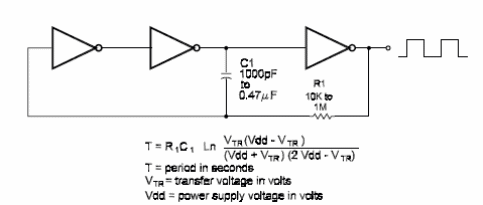### DatasheetsN° of component

## Three-Gate Ring Oscillator (CB106)

An oscillator that uses two gates is not suitable for critical applications, as it tends to pick up noise from the power-supply line. This noise can affect the output, producing deformed signals. A better type for these applications is the threegate ring oscillaton It uses three inverters insbead of only two. Figure bellow shows the basic configuration for a three-gate ring oscillator using inverters [or any other function wired as inverter]. The frequency depends on R1 and C1 Using the values shown in the figure, the circuit will operate in the audio range. The lowest value recommended for C1 is 47 pF and is 19k ohm for R1. The frequency for this circuit can be calculated by using the formula in the diagram.Three~Gate Ring Oscillator